# Handling Domains with Different Materials¶

Solving PDEs in domains made up of different materials is a frequently encountered task. In FEniCS, these kind of problems are handled by defining subdomains inside the domain. The subdomains may represent the various materials. We can thereafter define material properties through functions, known in FEniCS as mesh functions, that are piecewise constant in each subdomain. A simple example with two materials (subdomains) in 2D will demonstrate the basic steps in the process. .. Later, a multi-material

## Working with Two Subdomains¶

Suppose we want to solve

(1)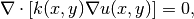in a domain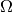consisting of two subdomains where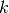takes on a different value in each subdomain. For simplicity, yet without loss of generality, we choose for the current implementation the domain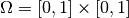and divide it into two equal subdomains,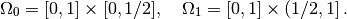We define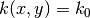in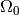and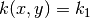in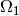, where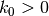and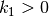are given constants. As boundary conditions, we choose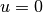at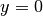,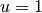at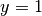, and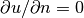at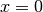and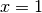. One can show that the exact solution is now given byAs long as the element boundaries coincide with the internal boundary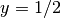, this piecewise linear solution should be exactly recovered by Lagrange elements of any degree. We use this property to verify the implementation.

Physically, the present problem may correspond to heat conduction, where the heat conduction inis ten times more efficient than in. An alternative interpretation is flow in porous media with two geological layers, where the layers’ ability to transport the fluid differs by a factor of 10.

## Implementation (3)¶

The new functionality in this subsection regards how to define the subdomainsand. For this purpose we need to use subclasses of class SubDomain, not only plain functions as we have used so far for specifying boundaries. Consider the boundary function

def boundary(x, on_boundary):
tol = 1E-14
return on_boundary and abs(x) < tol


for defining the boundary. Instead of using such a stand-alone function, we can create an instance (or object) of a subclass of SubDomain, which implements the inside method as an alternative to the boundary function:

class Boundary(SubDomain):
def inside(self, x, on_boundary):
tol = 1E-14
return on_boundary and abs(x) < tol

boundary = Boundary()
bc = DirichletBC(V, Constant(0), boundary)


A word about computer science terminology may be used here: The term instance means a Python object of a particular type (such as SubDomain, Function FunctionSpace, etc.). Many use instance and object as interchangeable terms. In other computer programming languages one may also use the term variable for the same thing. We mostly use the well-known term object in this text.

A subclass of SubDomain with an inside method offers functionality for marking parts of the domain or the boundary. Now we need to define one class for the subdomainwhere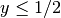and another for the subdomainwhere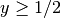:

class Omega0(SubDomain):
def inside(self, x, on_boundary):
return True if x <= 0.5 else False

class Omega1(SubDomain):
def inside(self, x, on_boundary):
return True if x >= 0.5 else False


Notice the use of <= and >= in both tests. For a cell to belong to, e.g.,, the inside method must return True for all the vertices x of the cell. So to make the cells at the internal boundarybelong to, we need the test x >= 0.5.

The next task is to use a MeshFunction to mark all cells inwith the subdomain number 0 and all cells inwith the subdomain number 1. Our convention is to number subdomains as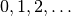.

A MeshFunction is a discrete function that can be evaluated at a set of so-called mesh entities. Examples of mesh entities are cells, facets, and vertices. A MeshFunction over cells is suitable to represent subdomains (materials), while a MeshFunction over facets is used to represent pieces of external or internal boundaries. Mesh functions over vertices can be used to describe continuous fields.

Since we need to define subdomains ofin the present example, we must make use of a MeshFunction over cells. The MeshFunction constructor is fed with three arguments: 1) the type of value: 'int' for integers, 'uint' for positive (unsigned) integers, 'double' for real numbers, and 'bool' for logical values; 2) a Mesh object, and 3) the topological dimension of the mesh entity in question: cells have topological dimension equal to the number of space dimensions in the PDE problem, and facets have one dimension lower. Alternatively, the constructor can take just a filename and initialize the MeshFunction from data in a file.

We start with creating a MeshFunction whose values are non-negative integers ('uint') for numbering the subdomains. The mesh entities of interest are the cells, which have dimension 2 in a two-dimensional problem (1 in 1D, 3 in 3D). The appropriate code for defining the MeshFunction for two subdomains then reads

subdomains = MeshFunction('uint', mesh, 2)
# Mark subdomains with numbers 0 and 1
subdomain0 = Omega0()
subdomain0.mark(subdomains, 0)
subdomain1 = Omega1()
subdomain1.mark(subdomains, 1)


Calling subdomains.array() returns a numpy array of the subdomain values. That is, subdomain.array()[i] is the subdomain value of cell number i. This array is used to look up the subdomain or material number of a specific element.

We need a function k that is constant in each subdomainand. Since we want k to be a finite element function, it is natural to choose a space of functions that are constant over each element. The family of discontinuous Galerkin methods, in FEniCS denoted by 'DG', is suitable for this purpose. Since we want functions that are piecewise constant, the value of the degree parameter is zero:

V0 = FunctionSpace(mesh, 'DG', 0)
k  = Function(V0)


To fill k with the right values in each element, we loop over all cells (i.e., indices in subdomain.array()), extract the corresponding subdomain number of a cell, and assign the correspondingvalue to the k.vector() array:

k_values = [1.5, 50]  # values of k in the two subdomains
for cell_no in range(len(subdomains.array())):
subdomain_no = subdomains.array()[cell_no]
k.vector()[cell_no] = k_values[subdomain_no]


Long loops in Python are known to be slow, so for large meshes it is preferable to avoid such loops and instead use vectorized code. Normally this implies that the loop must be replaced by calls to functions from the numpy library that operate on complete arrays (in efficient C code). The functionality we want in the present case is to compute an array of the same size as subdomain.array(), but where the value i of an entry in subdomain.array() is replaced by k_values[i]. Such an operation is carried out by the numpy function choose:

help = numpy.asarray(subdomains.array(), dtype=numpy.int32)
k.vector()[:] = numpy.choose(help, k_values)


The help array is required since choose cannot work with subdomain.array() because this array has elements of type uint32. We must therefore transform this array to an array help with standard int32 integers.

Having the k function ready for finite element computations, we can proceed in the normal manner with defining essential boundary conditions, as in the section Multiple Dirichlet Conditions, and the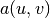and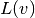forms, as in the section A Variable-Coefficient Poisson Problem. All the details can be found in the file mat2_p2D.py.

## Multiple Neumann, Robin, and Dirichlet Condition¶

Let us go back to the model problem from the section Multiple Dirichlet Conditions where we had both Dirichlet and Neumann conditions. The term v*g*ds in the expression for L implies a boundary integral over the complete boundary, or in FEniCS terms, an integral over all exterior facets. However, the contributions from the parts of the boundary where we have Dirichlet conditions are erased when the linear system is modified by the Dirichlet conditions. We would like, from an efficiency point of view, to integrate v*g*ds only over the parts of the boundary where we actually have Neumann conditions. And more importantly, in other problems one may have different Neumann conditions or other conditions like the Robin type condition. With the mesh function concept we can mark different parts of the boundary and integrate over specific parts. The same concept can also be used to treat multiple Dirichlet conditions. The forthcoming text illustrates how this is done.

Essentially, we still stick to the model problem from the section Multiple Dirichlet Conditions, but replace the Neumann condition atby a Robin condition: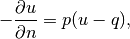where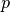and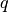are specified functions. The Robin condition is most often used to model heat transfer to the surroundings and arise naturally from Newton’s cooling law.

Since we have prescribed a simple solution in our model problem,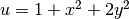, we adjustandsuch that the condition holds at. This implies that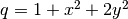andcan be arbitrary (the normal derivative at: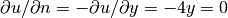).

Now we have four parts of the boundary: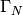which corresponds to the upper side,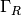which corresponds to the lower part,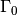which corresponds to the left part, and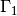which corresponds to the right part. The complete boundary-value problem reads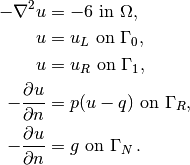The involved prescribed functions are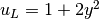,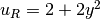,,is arbitrary, and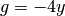.

Integration by parts of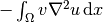becomes as usual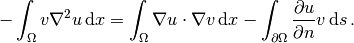The boundary integral vanishes on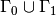, and we split the parts overandsince we have different conditions at those parts: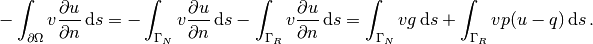The weak form then becomes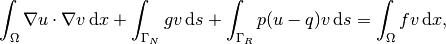We want to write this weak form in the standard notation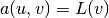, which requires that we identify all integrals with both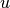and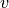, and collect these in, while the remaining integrals withand notgo into. The integral from the Robin condition must of this reason be split in two parts: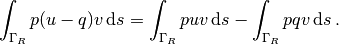We then haveA natural starting point for implementation is the dn2_p2D.py program in the directory stationary/poisson. The new aspects are

• definition of a mesh function over the boundary,
• marking each side as a subdomain, using the mesh function,
• splitting a boundary integral into parts.

Task 1 makes use of the MeshFunction object, but contrary to the section Implementation (3), this is not a function over cells, but a function over cell facets. The topological dimension of cell facets is one lower than the cell interiors, so in a two-dimensional problem the dimension becomes 1. In general, the facet dimension is given as mesh.topology().dim()-1, which we use in the code for ease of direct reuse in other problems. The construction of a MeshFunction object to mark boundary parts now reads

boundary_parts = \
MeshFunction("uint", mesh, mesh.topology().dim()-1)


As in the section Implementation (3) we use a subclass of SubDomain to identify the various parts of the mesh function. Problems with domains of more complicated geometries may set the mesh function for marking boundaries as part of the mesh generation. In our case, theboundary can be marked by

class LowerRobinBoundary(SubDomain):
def inside(self, x, on_boundary):
tol = 1E-14   # tolerance for coordinate comparisons
return on_boundary and abs(x) < tol

Gamma_R = LowerRobinBoundary()
Gamma_R.mark(boundary_parts, 0)


The code for theboundary is similar and is seen in dnr_p2D.py.

The Dirichlet boundaries are marked similarly, using subdomain number 2 forand 3 for:

class LeftBoundary(SubDomain):
def inside(self, x, on_boundary):
tol = 1E-14   # tolerance for coordinate comparisons
return on_boundary and abs(x) < tol

Gamma_0 = LeftBoundary()
Gamma_0.mark(boundary_parts, 2)

class RightBoundary(SubDomain):
def inside(self, x, on_boundary):
tol = 1E-14   # tolerance for coordinate comparisons
return on_boundary and abs(x - 1) < tol

Gamma_1 = RightBoundary()
Gamma_1.mark(boundary_parts, 3)


Specifying the DirichletBC objects may now make use of the mesh function (instead of a SubDomain subclass object) and an indicator for which subdomain each condition should be applied to:

u_L = Expression('1 + 2*x*x')
u_R = Expression('2 + 2*x*x')
bcs = [DirichletBC(V, u_L, boundary_parts, 2),
DirichletBC(V, u_R, boundary_parts, 3)]


Some functions need to be defined before we can go on with the a and L of the variational problem:

g = Expression('-4*x')
q = Expression('1 + x*x + 2*x*x')
p = Constant(100)  # arbitrary function can go here
u = TrialFunction(V)
v = TestFunction(V)
f = Constant(-6.0)


The new aspect of the variational problem is the two distinct boundary integrals. Having a mesh function over exterior cell facets (our boundary_parts object), where subdomains (boundary parts) are numbered as, the special symbol ds(0) implies integration over subdomain (part) 0, ds(1) denotes integration over subdomain (part) 1, and so on. The idea of multiple ds-type objects generalizes to volume integrals too: dx(0), dx(1), etc., are used to integrate over subdomain 0, 1, etc., inside.

The variational problem can be defined as

a = inner(grad(u), grad(v))*dx + p*u*v*ds(0)
L = f*v*dx - g*v*ds(1) + p*q*v*ds(0)


For the ds(0) and ds(1) symbols to work we must obviously connect them (or a and L) to the mesh function marking parts of the boundary. This is done by a certain keyword argument to the assemble function:

A = assemble(a, exterior_facet_domains=boundary_parts)
b = assemble(L, exterior_facet_domains=boundary_parts)


Then essential boundary conditions are enforced, and the system can be solved in the usual way:

for bc in bcs:
bc.apply(A, b)
u = Function(V)
U = u.vector()
solve(A, U, b)


The complete code is in the dnr_p2D.py file in the stationary/poisson directory.